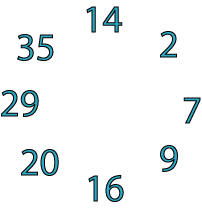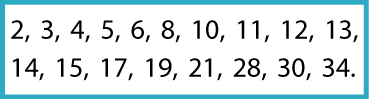## Wednesday, 27 April 2022

### Key Stage 2: Summer 1 - Maths Challenge 25.04.22

In the circle of numbers below each adjoining pair adds to make a square number:For example,

14+2=16,2+7=9,7+9=16

and so on.

Can you make a similar - but larger - cycle of pairs that each add to make a square number, using all the numbers in the box below, once and once only?